Know more about the Repeaters Batch Know more about the Repeaters Batch

# Centre of Mass of a Triangle

The centre of mass is also called the centre of gravity. The centre of mass of the triangle is the point at which the mass of the triangle will balance. To understand the “centre of mass” of a triangle, let us imagine balancing triangular cardboard on the pencil tip. The cardboard will balance on the pencil tip if it is placed at the centre of mass.

For an equilateral triangle centre of mass yc = h/3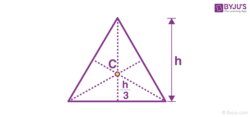## Methods to Find Centre of Mass of Triangle

Let us discuss how to determine the centre of mass of a triangle of unequal sides.

Method 1: Using intersecting meridians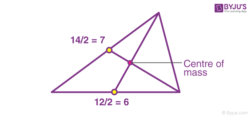Step 1: Calculate the midpoint of one of the sides of the triangle

Measure the length of the side and then divide it by 2 to get the midpoint of the triangle. Mark the point as A.

If the length of the side is 12 then the midpoint will be 12/2 = 6

Step 2: Calculate the midpoint of the second side of the triangle

Find the second side length and divide it into half. If the length of the side is 14 then the midpoint of that side is 14/2 = 7. Mark the point as B.

Step 3: A line must be drawn from the midpoints to the opposite vertex.

The two lines drawn from the midpoints are the median of each side and the point at which the two sides of the triangle meet is the vertex.

Step 4: Mark the point where the medians meet

This point will be the centre of mass of the triangle.

Method 2: Using 2:1 ratio method

Step 1: Draw a median of the triangle

Median is the line drawn from the midpoint of one the sides of the triangle to the opposite vertex.

Step 2: Find the length of the meridian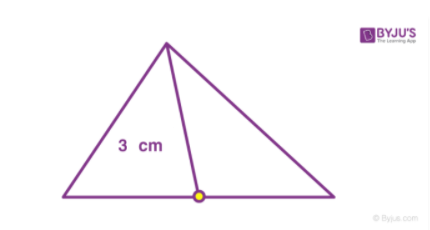Step 3: Divide the length of the meridian by 3

If the length was measured as 3, divide it by 3

3/3 = 1. So the length of ⅓ of the meridian is 1

Step 4: Mark the point on the ⅓ of the meridian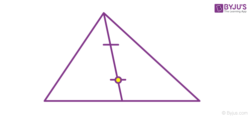This point is the centre of mass of the triangle.

Method 3: Using average coordinates

Step 1: Find the coordinates of all three vertices

The triangle must be drawn on the graph and mark the coordinates. The coordinates should be marked as (x,y).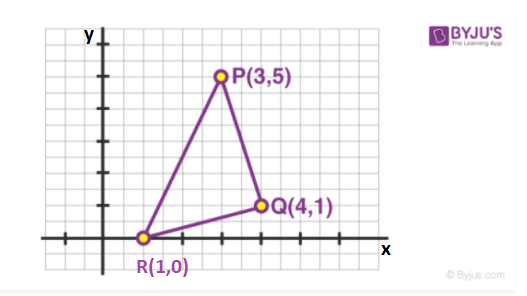Step 2: Add the values of x coordinates

For example,if the three coordinates are 3 ,4 ,1 then 3+4+1= 8.

Step 3: Add the values of y coordinates

For example, if the three coordinates are 5,1,0 then 5+1+0 = 6

Step 4: Find the average of x and y coordinates

The average of x coordinates is 8 therefore the average is 8/3. The average of y coordinates is 6/3 = 2.

Step 5: Plot the points on the graph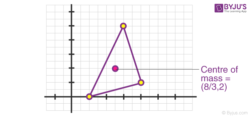The point marked is the centre of mass of the triangle.

## Frequently Asked Questions on Centre of Mass of a Triangle

### What is the position of the triangular lamina’s centre of mass?

The intersection of the medians is where a triangular lamina’s centre of mass is located. As a result, it is the triangle’s centroid.

### What is a lamina?

A closed two-dimensional flat surface with mass and surface density. The geometric centroid of a lamina is its centre of mass.

### What is the triangle’s centroid?

The point of intersection of a triangle’s medians is its centroid.

Test your knowledge on Centre Of Mass Of A Triangle